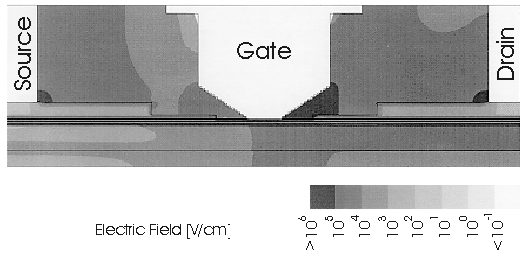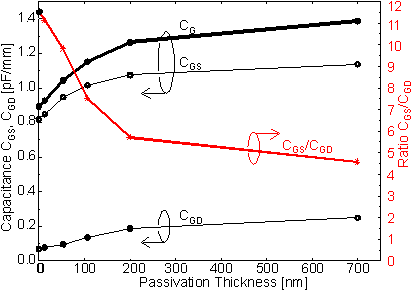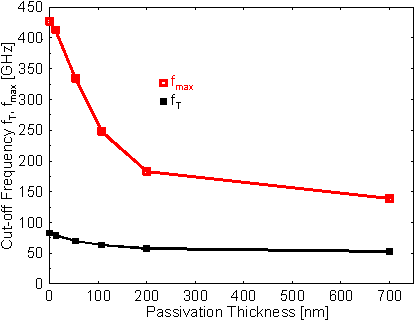Next: 6.2.3.4 Dependence of CG on T-Gate Cross Section Up: 6.2.3 RF Characteristics Previous: 6.2.3.2 Contributions to the Gate Capacitance

6.2.3.3 Dependence of CG on Passivation Thickness

As shown in (62) the second term of CG depends linearly on er. Unfortunately, there exist no suitable passivation materials with er significantly smaller than 7. A way to practically reduce the average er in the space between semiconductor surface and contacts is to decrease the thickness of the dielectric coating such that this space is partially filled with air. This was done experimentally in  by selectively etching away part of the passivation.

The change in CG strongly depends on the electric field E in the space where the passivation is removed, (i. e., er is changed from 7 to 1).  Figure 6.33 shows the spatial distribution of E for the HEMT with LG = 220 nm at a bias VDS = 2.0 V, VGS = 0.4 V. The highest field appears at the drain-sided end of the gate footprint and decreases strongly towards the top of the gate contact. Therefore, only a moderate decrease of CG is expected when only the top part of the passivation is removed.Figure 6.33 Electric field of the simulated HEMT in true scale at VDS = 2.0 V, VGS = 0.4 V.

This is quantitatively shown in  Figure 6.34. Simulations were performed for partially removed passivation, i. e. er2 =  7 and er1 = 1, where the interface between both regions is horizontal as indicated in  Figure 6.21. The capacitance CG is reduced by about 120 fF/mm when the passivation is thinned to a residual thickness of 200 nm (passivation surface on a level with cap surface). The same decrease of CG would be obtained by a reduction of LG to about 40 nm, as can be deduced from  Figure 6.32. An even larger reduction of about 500 fF/mm is calculated for complete omission of the dielectric layer (case er  = 1 in Figure 6.32). For comparison, the term LG A3 in (62) has a value of about 700 fF/mm for the HEMT with LG = 220 nm.Figure 6.34 Capacitances CG, CGS, CGD, and the ratio CGS /CGD as a function of passivation thickness.

Among the various capacitances that characterize a transistor, we have up to now only considered CG and its influence on fT. However, as a figure of merit for RF performance, the maximum frequency of oscillation fmax is even more significant than fT. Prerequisite for high fmax is a large ratio CGS/CGD.

In  Figure 6.34, the calculated quantities CGS, CGD and CGS/CGD are shown as a function of passivation thickness. With decreasing thickness, CGD decreases more rapidly than CGS, in our particular case especially for thicknesses below 200 nm. The ratio CGS/CGD accordingly rises dramatically.

fmax can be calculated according to (11) under the assumption that only CGS and CGD are changed by the passivation thickness. In reality the interface charge density on the semiconductor surface is strongly increased when the passivation gets very thin or is even removed completely. An increased interface charge density reduces the current for high VGS and thus deteriorates gm . Additional drawbacks of removing the passivation completely are low yield in the manufacturing process and serious reliability problems.

In  Figure 6.35 the calculated fT and fmax is shown. fT increases strongly from 53 GHz to about 80 GHz but fmax even increases from about 140 GHz by a factor of 3. As a more practical case we can consider the previous case of a residual passivation thickness of 200 nm. It can be expected that fT increases from 53 GHz to 60 GHz and fmax from 140 GHz to 180 GHz.Figure 6.35 Simulated gate capacitance CG vs. gate length LG for two different er at VDS = 2.0 V, ID = 160 mA/mm.

This result leads to two important conclusions: First, for high fmax, a thin passivation is very favorable, but, secondly, in this regime of small parasitic capacitances, CGS/CGD is subject to very rapid changes caused by very small process variations. Consequently, it is extremely difficult to achieve tight control over fmax, and hence the gain.Next: 6.2.3.4 Dependence of CG on T-Gate Cross Section Up: 6.2.3 RF Characteristics Previous: 6.2.3.2 Contributions to the Gate Capacitance

Helmut Brech
1998-03-11